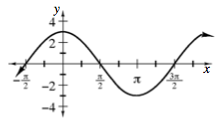Home > CCA2 > Chapter 8 > Lesson 8.3.2 > Problem8-146

8-146.
1. Sketch the graph of each equation below. Homework Help ✎

1. y = 3 sin (x +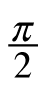)

2. y = −2 sin (4x)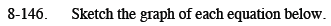$\text{locator point} = (- \frac{\pi}{2} , 0)$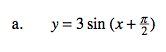$\text{b = 4, period} = \frac{2 \pi}{4} = \frac{\pi}{2}$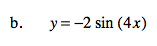b = 1, period = 2π

amplitude = 3

no vertical shift, midline is y = 0.